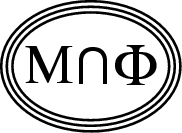Back to
OP page

Combined
List

List by
Contributors

# OPTIMAL FLUX FOR THE QUARTER-FILLED BAND

### Further technical details are in the following TeX document.

\magnification=\magstep1
\baselineskip=3ex

{\bf THE OPTIMAL FLUX FOR THE QUARTER-FILLED BAND'.} Consider a large square lattice $\Lambda$ with $|\Lambda|$ sites and with periodic boundary conditions. We assume that $|\Lambda|$ is divisible by 4. Now consider the hopping matrix $T$ on $\Lambda$, but with a magnetic field, i.e., $T_{x,y} =\exp[i\theta (x,y)]$ if $x,y$ are neighboring sites of $\Lambda$ and $T_{x,y} =0$ otherwise. The real numbers $\theta (x,y)$ are arbitrary, except for the hermiticity condition that $\theta (x,y) =- \theta (y,x)$. Let $\lambda_1\leq \lambda_2\leq \lambda_3\leq ... \leq \lambda_{|\Lambda|}$ denote the eigenvalues of $T$. It is easy to prove that these eigenvalues depend only on the fluxes, namely the sum of the $\theta (x,y)$ around the elementary small squares (or plaquettes) of $\Lambda$. With $E = \sum_{j=1}^{ |\Lambda|/4} \lambda_j$ denoting the sum of the lowest one quarter of the eigenvalues, our goal is to find the choice of fluxes that minimize $E$. It has been conjectured that the optimum choice is when the flux through each elementary square is $\pi / 2$. (Note: The corresponding problem for the half-filled band is known to have the optimum flux $= \pi$, even when a Hubbard type on-site interaction is included; E. H. Lieb, Phys. Rev. Lett. {\it 73}, 2158-2161 (1994).)